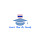## Monday, May 25, 2020

### Lesson 10 How to use vowels

How to use vowels

There are 5 ways to use vowels in summary.

1. Stable form
Meaning that the form must be clearly written such as กะบะ (Ga Ba), กะปิ (Ga Pi)

2. Reduce form
Meaning that you don't have to write vowels to appear or appear only partially But must pronounce to match the reduced vowel form, there are 2 types as follows

2.2 Reduce all
น (Nor)        +     โ-ะ (oh,o)         +     ก (Gor)    =    นก (Nog)
ม (Mor)       +      โ-ะ (oh,o)       +     ด (Dor)     =    มด (Mod)
ก (Gor)        +     -อ (aw, or)       +     ร (Ror)     =    กร (Gorn)
จ (Jor)          +     -อ (aw, or)       +     ร (Ror)     =    จร (Jorn)

2.3 Partially reduced
Meaning that the vowel cannot be completely reduced. Leaving only some parts of the form full enough as a mark to notice that it is not the same as other forms as follows

ก (Gor)      +      เ-อ (er,oe)           +     ย (Yor)     =    เกย (Goey)
ก (Gor)      +     -ัว (aw, or)           +     น (Nor)     =    กวน (Gawn)

3. Transform
Meaning that to convert the original form to change into another form as follows

ก (Gor)        +     -ะ (a)                     +     น (Nor)           =    กัน (Gan)
ก (Gor)       +      เ-ะ (ay)                  +    ง (Ngor)           =    เก็ง (Geng)
ข (Khor)      +    แ-ะ (ae)                  +     ง (Ngor)          =    แข็ง (Khaeng)
ด (Dor)        +     เ-อ (er, oe, ur)      +     น (Nor)            =    เดิน (Durn)

4. Cut form
Meaning that  to cut the vowels  " ะ " that are the front vowels of the words that come from Pali and Sanskrit And do not have to pronounce the vowel cut (Meaning both images and sounds) as follows

อนุช (Anooch) - นุช (Nooch)             อดิเรก (Adireg) - ดิเรก (Direg)

5. Fill form

ม (Khor)      +    -ึ (eu)      add     อ (Or)          =    มือ (Meu)
ค (Dor)        +    -ึ (eu)      add     อ (Or)          =    คือ (Keu)

1.2.